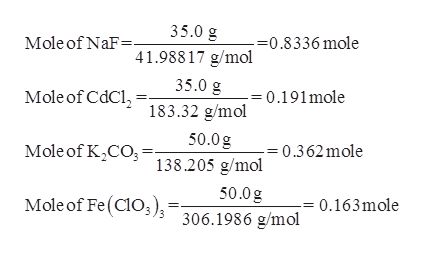# What volume of each of the following solutions contains the indicated amount of dissolved solute?35.0 g solute in 0.275 M NaF35.0 g solute in 0.275 M CdCl250.0 g solute in 0.418 M K2CO350.0 g solute in 0.418 M Fe(ClO3) 3

Question
44 views

What volume of each of the following solutions contains the indicated amount of dissolved solute?

35.0 g solute in 0.275 NaF

35.0 g solute in 0.275 M CdCl2

50.0 g solute in 0.418 M K2CO3

50.0 g solute in 0.418 M Fe(ClO3) 3

check_circle

Step 1

Molarity:

Dividing moles of solute by the volume of solution in Liter.

Step 2

Mole:

Dividing taken mass substance by molar mass of substance.

Step 3

Find the mole of solute that is present in give mass of solutes,

Molar mass of NaF is 41.98817 g/mol

Molar mass of CdCl2 is 183.32 g/mol

Molar m...help_outlineImage Transcriptionclose35.0 g Mole of NaF= -=0.8336 mole 41.98817 g/mol 35.0 g Mole of CdCl, = 0.191mole 183.32 g/mol 50.0g = 0.362 mole Mole of K,CO; : 138.205 g/mol 50.0g Mole of Fe (Cl03), 0.163mole 306.1986 g/mol fullscreen

### Want to see the full answer?

See Solution

#### Want to see this answer and more?

Solutions are written by subject experts who are available 24/7. Questions are typically answered within 1 hour.*

See Solution
*Response times may vary by subject and question.
Tagged in

### General Chemistry